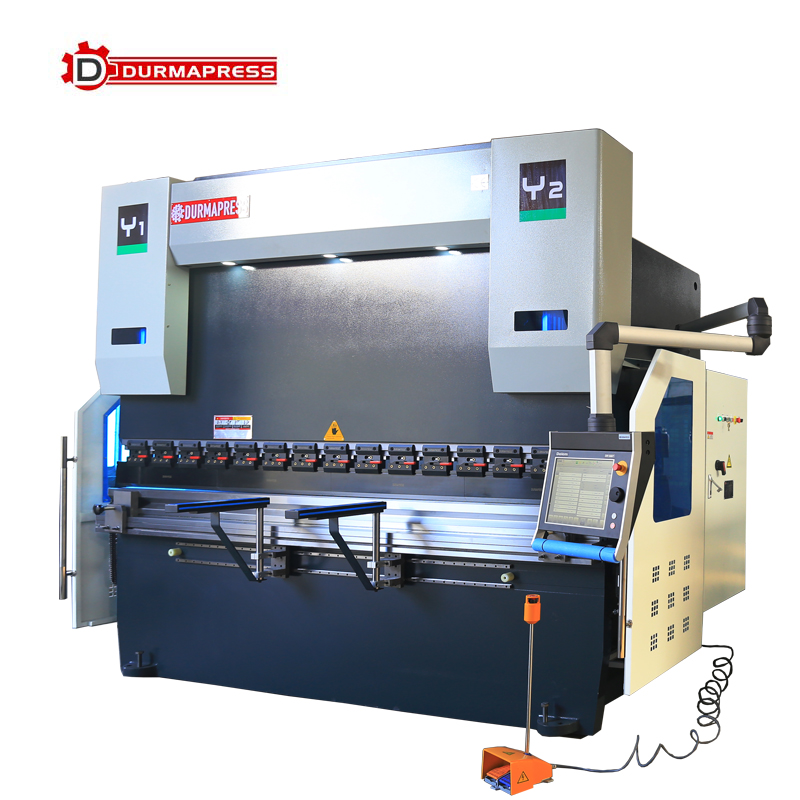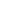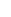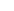E-mail Us： info@durmapress.com

# Bending a knife, bending press brake machine panel to set what parameters

Views:2     Author:DURMAPRESS     Publish Time: 2021-06-08      Origin:DURMAPRESS InquireIf a bending press brake machine workpiece with a single elbow is shown in the figure below, the operation process in the manual machining page is as follows:

It is not difficult to see from the figure: the thickness of the workpiece plate is 2mm, single-end flanging height is 20mm.In addition, it is assumed that the sheet material is Q235-A and the total processing width is 2000mm.

For some information in the figure above, please select the right upper and lower molds and input the information into the corresponding parameters of the CNC system.

All of these parameters can be programmed independently bending press brake

Upper mold UP: select the corresponding upper mold from the memory of the upper mold for processing, just input the serial number of the upper mold.(No. 5 is entered in the example)

Lower mould UN: select the corresponding lower mould from the memory of the lower mould for processing, just input the serial number of the lower mould.(The input in the example is No. 5) Material MA: Select the type of processed sheet material (input 1 in this example)1: Steel 4: Stainless steel

2: Aluminum 5: According to the definition of tensile strength and E-modulus set in the programming constants

Material MA: Select the type of processed sheet material (enter 1 in this example)

1: Steel 4: Stainless steel

2: Aluminum 5: According to the definition of tensile strength and E-modulus set in the programming constantsThickness TH: Thickness of processed sheet (input 2 in this example)

Length BL: width of processed sheet (enter 2000 in this example)

Bending mode BM: Bending mode (enter 0 in this example)

0= Free bending 10: Flat bending

1= bottom bending 11: flattening + bottom bending

Correct Ca= positive bending Angle

If 90 degrees is programmed and 91 degrees is actually processed, then the kernel is positive -1 degrees

If the programming 90 degrees, the actual processing is 89 degrees, then the kernel positive is +1 degrees

Angle a= bending Angle (enter 90 in this example)Y axis Y= programming or calculated Y axis value (by the numerical control system according to machine parameters, mold parameters, plate thickness automatically calculated the bending depth, with the enter key directly confirm the numerical control system calculated value;If the correction Angle is entered at the Angle CA, please use the Enter key to reconfirm the Y-axis value.) Speed conversion point Mutem = the position of the speed conversion point (the CNC system automatically calculates the speed conversion point of the slider according to the parameters of the machine tool, the mold parameters and the thickness of the plate, and directly confirm the value calculated by the CNC system with the Enter key).

Pressure P= in bending, hydraulic system output bending tonnage (numerical control system according to sheet thickness and tensile strength, die opening, bending width and other parameters automatically calculated.It can also be modified manually.)X-axis X-rear stopper programming position (enter 18.1 in this example because the bending height in the figure is 20mm, including sheet thickness.In the process calculation of the automatic program, the numerical control system automatically calculates the positioning position of the X axis.

R-axis R- If the machine tool is equipped with additional axes such as X2, R, DF, etc., the programming value in the manual page is entered by the user according to needs) Opening Dy= the opening height of the slider. The height of the return trip of the slider is calculated from the height of the speed conversion.Speed S = bending (slider under slow speed, manually) decompression speed BS = unloading hydraulic system, the speed of the unloading (corresponding to the unloading distance, artificial entry, usually smaller than bending speed) delay time T = the holding time in bending the final points of (0.1 9.9 seconds) can modify the parallel Y2 = around cylinder deviation value is set.When positive, Y2 goes down a little bit, and when negative, Y2 goes down a little bit. This setting is used for free bending.

With no distractions and keep focus, we strive to improve our products, lead the way of innovation…
View More >0086 555 8327689+86 18325572889info@durmapress.com